Courses

# Number Representations Practice Quiz - 1

## 20 Questions MCQ Test Mock Test Series - Computer Science Engg. (CSE) GATE 2020 | Number Representations Practice Quiz - 1

Description
This mock test of Number Representations Practice Quiz - 1 for Computer Science Engineering (CSE) helps you for every Computer Science Engineering (CSE) entrance exam. This contains 20 Multiple Choice Questions for Computer Science Engineering (CSE) Number Representations Practice Quiz - 1 (mcq) to study with solutions a complete question bank. The solved questions answers in this Number Representations Practice Quiz - 1 quiz give you a good mix of easy questions and tough questions. Computer Science Engineering (CSE) students definitely take this Number Representations Practice Quiz - 1 exercise for a better result in the exam. You can find other Number Representations Practice Quiz - 1 extra questions, long questions & short questions for Computer Science Engineering (CSE) on EduRev as well by searching above.
*Answer can only contain numeric values
QUESTION: 1

### The 16  -bit 2` s complement representation of an integer is 1111  1111 1111    0101 ; its decimal representation is ______________.

Solution:

for 2's complement  1 111 1111 1111 0101 →2′scomplement→2′scomplement  1 000 0000 0000 1011
1st bit is same not involved in 2's complement same with 1's complement. since msb bit for sign.
Take one's complement and add 1 we get 11, and as it is negative number we get answer as -11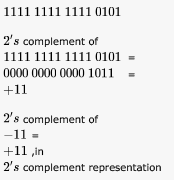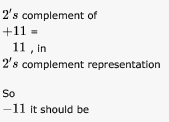*Answer can only contain numeric values
QUESTION: 2

### Let X be the number of distinct 16-bit integers in 2`s complement representation. Let Y be the number of distinct -bit integers in sign magnitude representation Then X  Y is______.

Solution:

For N bits, Distinct values represented in 2's complement is -2n-1 to 2n-1 -1
Distinct values represented in Signed Magnitude is -(2n-1 -1) to 2n-1 -1
Difference is 1.

*Answer can only contain numeric values
QUESTION: 3

### Define the value of r in the following: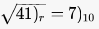Solution: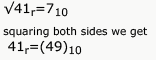4r+1=40+9
4r=48
r=12

*Answer can only contain numeric values
QUESTION: 4

Consider the number given by the decimal expression: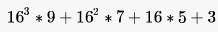The number of 1’s in the unsigned binary representation of the number is ______

Solution:

Hex representation of given no. is (9753)16
Its binary representation is (1001 0111 0101 0011)2 The no. of 1's is 9

*Answer can only contain numeric values
QUESTION: 5

Convert the following numbers in the given bases into their equivalents in the desired bases:

a. (110.101)2 = (x)10
b. (1118)10 = (y)H

Solution:

a. 1 * 22 + 1 * 21 + 0 * 20 + 1 * 2-1 + 0 * 2-2 + 1 * 2-3 = 6.625
b. 1118 mod 16 = 14, quotient = 69
69 mod 16 = 5, quotient = 4
4 mod 16 = 4.
Writing the mods in the reverse order (in hex) gives (45E)H
Both can be done using calculator also.

QUESTION: 6

The number of 1's in the binary representation of (3*4096 + 15*256 + 5*16 + 3) are:

Solution:

I suggest following approach , here we can clearly see that numbers are getting multiplied by powers of 16. So this is nothing but Hexadecimal number in disguise.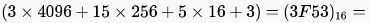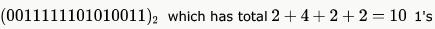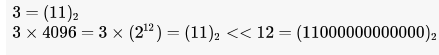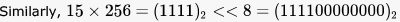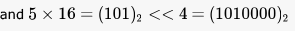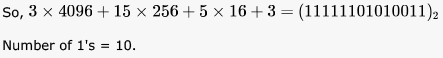QUESTION: 7

Consider the following floating-point number representation.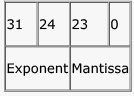The exponent is in 2’s complement representation and mantissa is in the sign magnitude representation. The range of the magnitude of the normalized numbers in this representation is

Solution:

Here, we are asked "magnitude" - so we just need to consider the mantissa bits.
Also, we are told "normalized representation"- so most significant bit of mantissa is always 1 (this is different from IEEE 754 normalized representation where this 1 is omitted in representation, but here it seems to be added on the right of decimal point as seen from options).
So, the maximum value of mantissa will be 23 1's where a decimal point is assumed before first 1. So, this value will be 1- 2-23.
Due to the 1 in normalized representation, the smallest positive number will be 1 followed by 23 0's which will be 2-1 = 0.5

Given that mantissa is sign magnitude representation so 1 bit for sign and remaining 23 bits for mantissa and  we have to find the range of mantissa in normalized form ....
so smallest will be = .100.....0(normalize form) which is 2^(-1) = .5
and for largest will be .111....1 which is 1-2^(-23) .
so range will be .5 to (1-2^(-23))

QUESTION: 8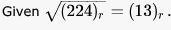The value of the radix r is:

Solution: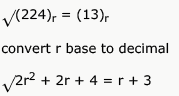2r2 + 2r + 4 = r2 + 6r+ 9
r2 - 4r -5 = 0
r2 -5r +r - 5 = 0
(r-5)(r+1)=0
r can not be -1
so r = 5 is correct answer

QUESTION: 9

The octal representation of an integer is (342)8. If this were to be treated as an eight-bit integer in an 8085 based computer, its decimal equivalent is

Solution: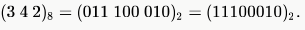If we treat this as an 8 bit integer, the first bit becomes sign bit and since it is "1", number is negative. Computer uses 2's complement representation for negative numbers and hence the decimal equivalent will be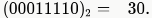QUESTION: 10

Suppose the domain set of an attribute consists of signed four digit numbers. What is the percentage of reduction in storage space of this attribute if it is stored as an integer rather than in character form?

Solution:

I assume byte addressable memory- nothing smaller than a byte can be used.
We have four digits. So, to represent signed 4 digit numbers we need 5 bytes- 4 for four digits and 1 for the sign (like -7354). So, required memory = 5 bytes
Now, if we use integer, the largest number needed to represent is 9999 and this requires 2 bytes of memory for signed representation.

So, memory savings while using integer is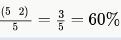*Multiple options can be correct
QUESTION: 11

Zero has two representations in

Solution:

Sign Magnitude
+0 = 0000
-0 = 1000
1's complement
+0 = 0000
-0 = 1111

QUESTION: 12

The number 43 in 2's complement representation is

Solution:

2's complement representation is not same as 2's complement of a number. In 2's complement representation positive integers are represented in its normal binary form while negative numbers are represented in its 2's complement form. So (c) is correct here.

QUESTION: 13

Consider the values of A = 2.0 x 1030, B = -2.0 x 1030 , C = 1.0, and the sequence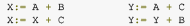executed on a computer where floating point numbers are represented with 32 bits. The values for X and Y will be

Solution:

Given 32 bits representation. So, the maximum precision can be 32 bits (In 32-bit IEEE representation, maximum precision is 24 bits but we take best case here). This means approximately 10 digits.
A = 2.0 * 1030, C = 1.0
So, A + C should make the 31st digit to 1, which is surely outside the precision level of A (it is 31st digit and not 31st bit).
So, this addition will just return the value of A which will be assigned to Y. So, Y + B will return 0.0 while X + C will return 1.0.
B choice.

QUESTION: 14

The 2's complement representation of (-539)10 in hexadecimal is

Solution:

539 = 512 + 16 + 8 + 2 + 1 = 29 + 24 + 23 + 21 + 20
= (1000011011)2
Now all answers have 12 bits, so we add two 0's at beginning = (001000011011)2
To convert to 2's complement invert all bits till the rightmost 1, which will be (110111100101)2
= (1101 1110 0101)2
= (DE5)16

QUESTION: 15

The decimal value 0.25

Solution:

1st Multiplication Iteration Multiply 0.25 by 2
0.25 x 2 = 0.50(Product)         Fractional part=0.50
Carry=0    (MSB)
2nd Multiplication Iteration
Multiply 0.50 by 2
0.50 x 2 = 1.00(Product)         Fractional part = 1.00
Carry = 1(LSB)
The fractional part in the 2nd iteration becomes zero and hence we stop the multiplication iteration.
Carry from the 1st multiplication iteration becomes MSB and  carry from 2nd iteration becomes LSB
So the Result is 0.01

QUESTION: 16

The 2's complement representation of the decimal value -15 is

Solution:

is the correct ans.In 2's complement representation, positive numbers are represented in simple binary form and negative numbers are represented in its 2's complement form. So, for -15, we have to complement its binary value -01111 and add a 1 to it, which gives 10001. Option D.

QUESTION: 17

Sign extension is a step in

Solution:

is the answer. Sign extension (filling the upper bits using the sign bit) is needed while increasing the number of bits for representing a number. For positive numbers, 0 is extended and for negative numbers 1 is extended.

QUESTION: 18

Solution:

is the answer. When a positive value and negative value are added overflow never happens.

QUESTION: 19

Assuming all numbers are in 2’s complement representation, which of the following numbers is divisible by 11111011?

Solution:

MSB of 2's compliment number has a weight of - 2^(n-1).
(Trick: (from reversing sign extension) just skip all leading 1's from MSB expect but 1, and then calculate the value as normal signed binary rep. ) so by calculating, we get the given number is -5 in decimal. and options are
a. -25
b. -28
c. -41
d. -37
Therefore it is clear that - 25 is divisible by - 5. so we can say that (a.) is correct.

QUESTION: 20

If 73x (in base-x number system) is equal to 54y (in base y-number system), the possible values of x and y are

Solution:

x * 7 + 3 = 5 * y + 4 => 7x = 5y + 1. Only option satisfying this is D.